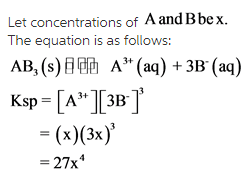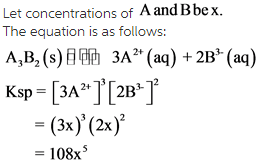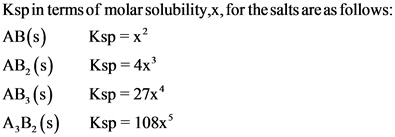# Ksp from molar solubility

By John

Express Ksp in terms of molar solubility, x, for a salt with a formula of
1. AB(s)
2. AB2(s)
3. AB3(s)
4. A3B2(s)

Concepts and reason
Solubility:
Solubility is defined as the maximum amount of solute dissolved in a given amount of solvent to make a saturated solution at a particular temperature.
Molar solubility:
The molar solubility is the number of moles of solute that dissolves in a litre of solution. It is expressed as mol/L or M (molarity).

Fundamentals
Solubility product: The equilibrium constant which defines the solubility of ionic substance in water is known as solubility product. The solubility product is denoted by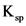.
For a saturated solution, the solubility product is the product of activities of the ions, each raised to its coefficients (powers).
Consider a general reaction: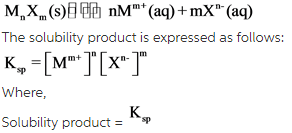The molar solubility of M ion = [M]
The molar solubility of X ion = [X]
The ions raised to the power= n and m

Let concentrations of A and B be X
The equation is as follows: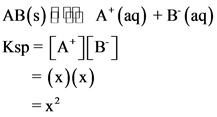The solubility equation of AB(S) is given above. The ionization of AB(S) gives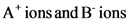.
The coefficient of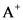ion is one and coefficient of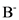ion is one. And theexpression for AB(S) is written with respect to the equation.